Courses

# RCC Design - 4

## 10 Questions MCQ Test GATE Civil Engineering (CE) 2022 Mock Test Series | RCC Design - 4

Description
This mock test of RCC Design - 4 for Civil Engineering (CE) helps you for every Civil Engineering (CE) entrance exam. This contains 10 Multiple Choice Questions for Civil Engineering (CE) RCC Design - 4 (mcq) to study with solutions a complete question bank. The solved questions answers in this RCC Design - 4 quiz give you a good mix of easy questions and tough questions. Civil Engineering (CE) students definitely take this RCC Design - 4 exercise for a better result in the exam. You can find other RCC Design - 4 extra questions, long questions & short questions for Civil Engineering (CE) on EduRev as well by searching above.
QUESTION: 1

### The purpose of reinforcement in prestressed concrete is

Solution:

Prestressed concrete import initial compressive stresses to counter tensile stresses due to loading.

Reinforcement bars are placed in a form and stressed or stretched with forces on each end of bars pulling them. When concrete is poured around the stressing bonds before pressure is released, it creates tension.

When the tension is released the steel tendency to try to resume its original shape and adds a compressive force to the concrete laterally, giving it strength to span distances.

QUESTION: 2

### Which of the following losses of prestress occurs only in pre-tensioning and not in post-tensioning?

Solution:

Elastic shortening:

• Pre - Tensioning:
• When the prestress is transmitted to the concrete member, there is contraction due to prestress. This contraction causes a loss of stretch in the wire.
• Post - Tensioning:
• No loss due to elastic deformation if all the wires are simultaneously tensioned. If the wires are successively tensioned, there will be loss of prestress due to elastic deformation of concrete.

Elastic shortening occurs only in pre-tensioning and may not occur in post-tensioning if all wires are tightened at same point of time.

QUESTION: 3

### As per IS 1343, The minimum clear spacing in case of single wires used in pre-tensioning system shall not be less than:

Solution:

As per clause 11.1.7.1 of IS 1343,

In case of single wires used in pre-tensioning system, the minimum clear spacing shall not be lesser than greater of the following:

1) 3 times the diameter of wire, and

2)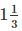times the maximum size of aggregate.

QUESTION: 4

In accordance with IS 1343: 1980, in the absence of data, the approximate value of shrinkage strain for the design of post-tensioning concrete member if the age of concrete at transfer is 3 days will be?

Solution:

According to 1343: 1980, clause 5.2.4.1,

The approximate value of shrinkage strain for design shall be assumed as follows:

(i) For Pre-tensioning = 0.0003
For Post − tensioning =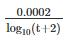For t = age of concrete at transfer (days)

At t = 3 days

Shrinkage strain will be: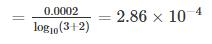QUESTION: 5

Match List – I (Post-tensioning system) with List – II (Arrangement of tendons in the duct) and select the correct answer using the codes given below the lists:

List - I

A. Freyssinte

B. Gifford-Udall

C. Lee-McCall

D. Magnel-Blaton

List – II

1. Single bars

2. Wires evenly spaced by perforated spacers

3. Horizontal rows of four wires spaced by metal grills

4. Wires spaced by helical wire core in annular spacer

Solution:

In Freyssinet system, high tension steel wires 5 mm to 8 mm diameter about 12 in number are arranged to form a group into a cable with a spiral spring inside. In the Gifford Udall system the wires are stressed and anchored one by one in a separate cylinder using small wedging grips called Udall grips.

In the Lee-Mc Call system, high tensile alloy steel bars are used as the prestressing tends.

In the Magnel Blaton system cable of rectangular section is provided. The wires are arranged with four wires per layer. The geometric pattern of the wires is maintains in the same from throughout the length of the cable by providing grills or spacers at regular intervals.

QUESTION: 6

An ordinary mild steel bar has been prestressed to working stress of 200 MPa. Young’s modulus of steel is 200 GPa. Permanent negative strain due to shrinkage and creep is 0.0008. How much is the effective stress left in steel?

Solution: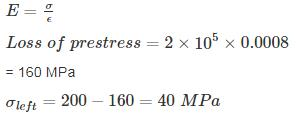QUESTION: 7

The percentage loss of prestress due to anchorage slip of 0.3 mm in a concrete beam of length 30 m which is post-tensioned by a tendon with initial stress of 1200 N/mm2 and modulus of elasticity equal to 2.1 × 105 N/mm2 is:

Solution:

Loss of prestress due to anchorage slip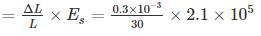= 2.1 N/mm2

loss of prestress =QUESTION: 8

A prestress concrete rectangular beam of size 300 mm × 900 mm is pre-stressed with an initial prestressing force of 700 kN at an eccentricity of 300 mm. Stress at the top beam section due to prestress alone in, N/mm2 is:

Solution: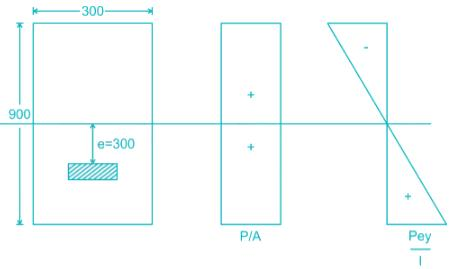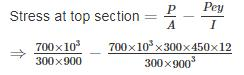⇒ 2.593 – 5.185

⇒ -2.592 N/mm2

QUESTION: 9

A concrete beam is prestressed by a cable carrying an initial prestress of 500 N/mm2. The percentage loss in prestressing due to shrinkage of concrete if the beam is pre-tensioned and post-tensioned. (Consider the age of concrete at transfer is 8 days, Es = 2 x 105 N/mm2)

Solution:

Shrinkage strain in concrete in pretension

ϵcs = 3 × 10-4

∴ loss in prestress, Δσ = ϵcs x Es

= 3 × 10-4 × 2 × 105

= 60 N/mm2

% loss in prestress = 60/500 × 100 = 12%
Shrinkage strain in post – tension,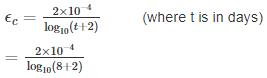= 2 × 10-4

∴ loss in prestress = ϵc × Es

= 2 × 10-4 × 2 × 105

= 40 N/mm2

% loss in presstress in post tension =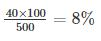QUESTION: 10

A prestressed beam of size 250 × 400 mm deep is prestressed by 14 tendons of 6 mm diameter. The cable is located at 150 mm from the bottom of the beam. If the initial prestress in the beam is 1250 N/mm2. The loss of prestress due to elastic deformation and creep of concrete respectively will be?

Assume:

M-40 Grade concrete

creep coefficient = 1.6

Modulus of elasticity = 2 × 105 MPa.

Neglect the effect due to dead load.

Solution:

Prestressing force (P) =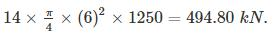Loss due to elastic shortening = mfc

fc = compressive stress at the level of steel

m = modular ration = Es/Ec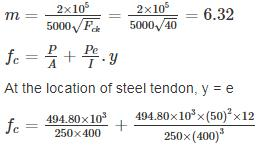fc = 4.948 + 0.9277

fc = 5.876 MPa

loss due to elastic shortening = 6.32 × 5.876

= 37.13 MPa.

Loss due to creep of concrete = mϕfc

ϕ = creep coefficient = 1.6

loss due to creep of concrete = 6.32 × 1.6 × 5.876

= 59.42 MPa.

• RCC